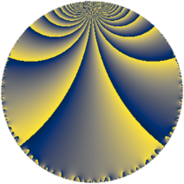Properties

 Label 37.4.fLevel $37$ Weight $4$ Character orbit 37.f Rep. character $\chi_{37}(7,\cdot)$ Character field $\Q(\zeta_{9})$ Dimension $54$ Newform subspaces $1$ Sturm bound $12$ Trace bound $0$

Related objects

Defining parameters

 Level: $$N$$ $$=$$ $$37$$ Weight: $$k$$ $$=$$ $$4$$ Character orbit: $$[\chi]$$ $$=$$ 37.f (of order $$9$$ and degree $$6$$) Character conductor: $$\operatorname{cond}(\chi)$$ $$=$$ $$37$$ Character field: $$\Q(\zeta_{9})$$ Newform subspaces: $$1$$ Sturm bound: $$12$$ Trace bound: $$0$$

Dimensions

The following table gives the dimensions of various subspaces of $$M_{4}(37, [\chi])$$.

Total New Old
Modular forms 66 66 0
Cusp forms 54 54 0
Eisenstein series 12 12 0

Trace form

 $$54 q - 9 q^{2} - 12 q^{3} + 9 q^{4} + 6 q^{5} - 12 q^{6} + 96 q^{7} + 18 q^{8} + 42 q^{9} + O(q^{10})$$ $$54 q - 9 q^{2} - 12 q^{3} + 9 q^{4} + 6 q^{5} - 12 q^{6} + 96 q^{7} + 18 q^{8} + 42 q^{9} - 63 q^{10} - 135 q^{11} - 315 q^{12} + 258 q^{13} - 15 q^{14} + 108 q^{15} - 87 q^{16} - 72 q^{17} - 660 q^{18} + 156 q^{19} + 636 q^{20} - 240 q^{21} + 63 q^{22} - 255 q^{23} + 87 q^{24} + 618 q^{25} - 417 q^{26} - 1095 q^{27} - 483 q^{28} - 63 q^{29} - 540 q^{30} - 708 q^{31} + 2502 q^{32} + 339 q^{33} + 633 q^{34} + 192 q^{35} + 2364 q^{36} + 1812 q^{37} + 210 q^{38} + 834 q^{39} - 465 q^{40} + 1050 q^{41} - 2061 q^{42} + 1056 q^{43} + 684 q^{44} - 1479 q^{45} - 3570 q^{46} - 1635 q^{47} - 1875 q^{48} - 2196 q^{49} + 795 q^{50} + 1047 q^{51} - 1389 q^{52} - 420 q^{53} + 1335 q^{54} - 621 q^{55} + 5403 q^{56} + 2196 q^{57} - 4293 q^{58} - 2130 q^{59} - 6612 q^{60} - 3222 q^{61} - 240 q^{62} - 1143 q^{63} - 2088 q^{64} - 3330 q^{65} + 5007 q^{66} + 522 q^{67} - 3054 q^{68} + 7227 q^{69} + 9978 q^{70} - 1656 q^{71} + 381 q^{72} + 1968 q^{73} + 7515 q^{74} + 6996 q^{75} + 2229 q^{76} + 843 q^{77} + 1329 q^{78} - 2508 q^{79} + 10992 q^{80} + 723 q^{81} + 141 q^{82} + 3048 q^{83} - 4920 q^{84} - 4365 q^{85} - 5496 q^{86} - 663 q^{87} + 6438 q^{88} - 2436 q^{89} - 17880 q^{90} - 7794 q^{91} - 11196 q^{92} + 3564 q^{93} - 708 q^{94} - 5922 q^{95} - 9663 q^{96} - 4359 q^{97} - 1725 q^{98} + 9399 q^{99} + O(q^{100})$$

Decomposition of $$S_{4}^{\mathrm{new}}(37, [\chi])$$ into newform subspaces

Label Dim. $$A$$ Field CM Traces $q$-expansion
$a_{2}$ $a_{3}$ $a_{5}$ $a_{7}$
37.4.f.a $54$ $2.183$ None $$-9$$ $$-12$$ $$6$$ $$96$$